# Elaborator Reflection - Identity Example¶

 This example of elaborator reflection steps through this metaprogram that generates the identity function: %language ElabReflection idNat : Nat -> Nat idNat = %runElab (do intro {{x}} fill (Var {{x}}) solve) 
 At the beginning of executing the elaboration script, the initial state consists of a single hole of type Nat -> Nat. As a first approximation, the state consists of a term with holes in it, an indicator of which hole is focused, a queue of the next holes to focus on, and miscellaneous information like a source of fresh names. The intro tactic modifies this state, replacing the focused hole with a lambda and focusing on the lambda’s body.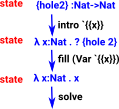The following is a walkthough looking at the state after each tactic:

 Start with the type signature like this: %language ElabReflection idNat : Nat -> Nat idNat = %runElab (do  In order to investigate how the program works this table shows the proof state at each stage as the tactics are applied. So here is the proof state at the start: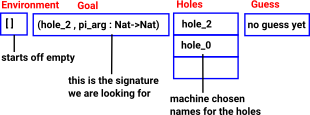This table shows the hole types and what they depend on. The aim is to illustrate the types by analogy with proofs, as a line with the premises above it and the conclusion below it.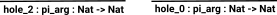The term is: ?{hole_0} ≈ ? {hole_2} . {hole_2} . {hole_0} It is possible to read the state from the script by calling getEnv, getGoal and getHoles. The output of these calls contain structures with TT code. To show the results I hacked this: my code . TT code is not really designed to be readable by humans, all the names are fully expanded, everything has a type down to universes (type of types). This is shown here to illustrate the information available. getEnv=[] getGoal=(hole_2, __pi_arg:(Nat.["Nat", "Prelude"]:{ type constructor tag=8 number=0}.Type:U=(20:./Prelude/Nat.idr)->. {name ref{type constructor tag=8 number=0}Nat.["Nat","Prelude"]: Type:U=(20:./Prelude/Nat.idr) }) }) getHoles=[{hole_2},{hole_0}]  getGuess error no guess Introduce a lambda binding around the current hole and focus on the body. intro {{x}} The state now looks like this: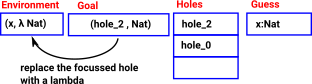The hole types now looks like this: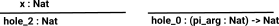The term now looks like this: ?{hole_0} ≈ λ x . ? {hole_2} . {hole_2} . {hole_0} Again we can check the state by calling getEnv, getGoal and getHoles: see my code  getEnv=[(x, {λ (Nat.["Nat", "Prelude"]:{ type constructor tag=8 number=0}). Type:U=(20:./Prelude/Nat.idr) })] getGoal=(hole_2, {name ref{type constructor tag=8 number=0} Nat.["Nat","Prelude"]:Type:U=(20:./Prelude/Nat.idr) }) getHoles=[{hole_2},{hole_0}]  getGuess error no guess Place a term into a hole, unifying its type fill (Var {{x}}) The state still looks like this:The hole types now looks like this: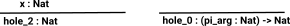The term now looks like this: ?{hole_0} ≈ λ x . ?{hole_2} ≈ x . {hole_2} . {hole_0} Again we can check the state by calling getEnv, getGoal and getHoles: see my code  getEnv=[(x, {λ (Nat.["Nat", "Prelude"]: {type constructor tag=8 number=0}). Type:U=(20:./Prelude/Nat.idr) })] getGoal=(hole_2, {name ref{type constructor tag=8 number=0} Nat.["Nat","Prelude"]:Type:U=(20:./Prelude/Nat.idr) }) getHoles=[{hole_2}, {hole_0}]  getGuess {name ref bound x: {name ref{type constructor tag=8 number=0} Nat.["Nat","Prelude"]:Type:U=(20:./Prelude/Nat.idr) } }  Substitute a guess into a hole. solve The hole types now looks like this: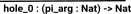The term now looks like this: ?{hole_0} ≈ λ x . x . {hole_0} getEnv getGoal getHoles getEnv=[] getGoal=(hole_0, __pi_arg:(Nat.["Nat", "Prelude"]:{ type constructor tag=8 number=0}. Type:U=(20:./Prelude/Nat.idr) ->.{name ref {type constructor tag=8 number=0} Nat.["Nat","Prelude"]:Type:U=(20:./Prelude/Nat.idr) }) }) getHoles=[{hole_0}]  getGuess x:({λ (Nat.["Nat", "Prelude"]:{ type constructor tag=8 number=0}). Type:U=(20:./Prelude/Nat.idr) }.{ name ref bound x:{name ref {type constructor tag=8 number=0} Nat.["Nat","Prelude"]:Type:U=(20:./Prelude/Nat.idr) } }) }